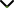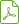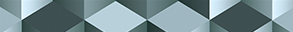# Fixed Point Theorems with Applications to the Solvability of Operator Equations and Inclusions on Function Spaces

Publishing date
01 May 2015
Status
Published
12 Dec 2014

Guest Editors

1King Abdulaziz University, Jeddah, Saudi Arabia

2University of Valencia, Valencia, Spain

4Universita degli Studi di Palermo, Palermo, Italy

# Fixed Point Theorems with Applications to the Solvability of Operator Equations and Inclusions on Function Spaces

## DescriptionFixed point theory is an interdisciplinary topic which provides insight and powerful tools for the solvability of central problems in many areas of current interest in mathematics as well as the other quantitative sciences, such as engineering, biology, economics, etc.

In fact, the existence and stability of solutions for linear and nonlinear problems are commonly translated into fixed point equivalent formulations, for example, the existence of solutions to elliptic partial differential equations, the existence of closed periodic orbits in dynamical systems, and, more recently, the existence of answer sets in logic programming. The objective of this special issue is to report the latest advancements in fixed point theory on abstract spaces (Banach spaces, locally convex spaces, metric spaces, etc.) and their applications to the solvability of nonlinear operator equations, which include ordinary differential equations, partial differential equations, functional differential equations, integral equations, integrodifferential equations, fractional differential equations, difference equations, etc.

The solvability of these equations is usually investigated in specific function spaces. The choice of the appropriate fixed point theorem and the use of specific properties of the underlying function space can have a significant impact on the solvability of these equations.

We aim to provide a platform for researchers to promote, share, and discuss various new issues and developments in this area.

Potential topics include, but are not limited to:

• Fixed point theorems in Banach spaces (for the weak and the strong topology)
• Fixed point theorems in Banach algebras
• Fixed point theorems in locally convex spaces
• Fixed point theorems in function spaces
• Operator equations in function spaces
• Operator inclusions in function spaces
• Evolution equations in function spaces
• Stochastic equations in function spacesDownload Special IssueJournal metrics
See full report
Acceptance rate33%
Submission to final decision52 days
Acceptance to publication26 days
CiteScore2.400
Journal Citation Indicator1.150
Impact Factor1.281Author guidelinesEditorial boardDatabases and indexing

Article of the Year Award: Outstanding research contributions of 2021, as selected by our Chief Editors. Read the winning articles.